## Prof. Dr. Moritz Weber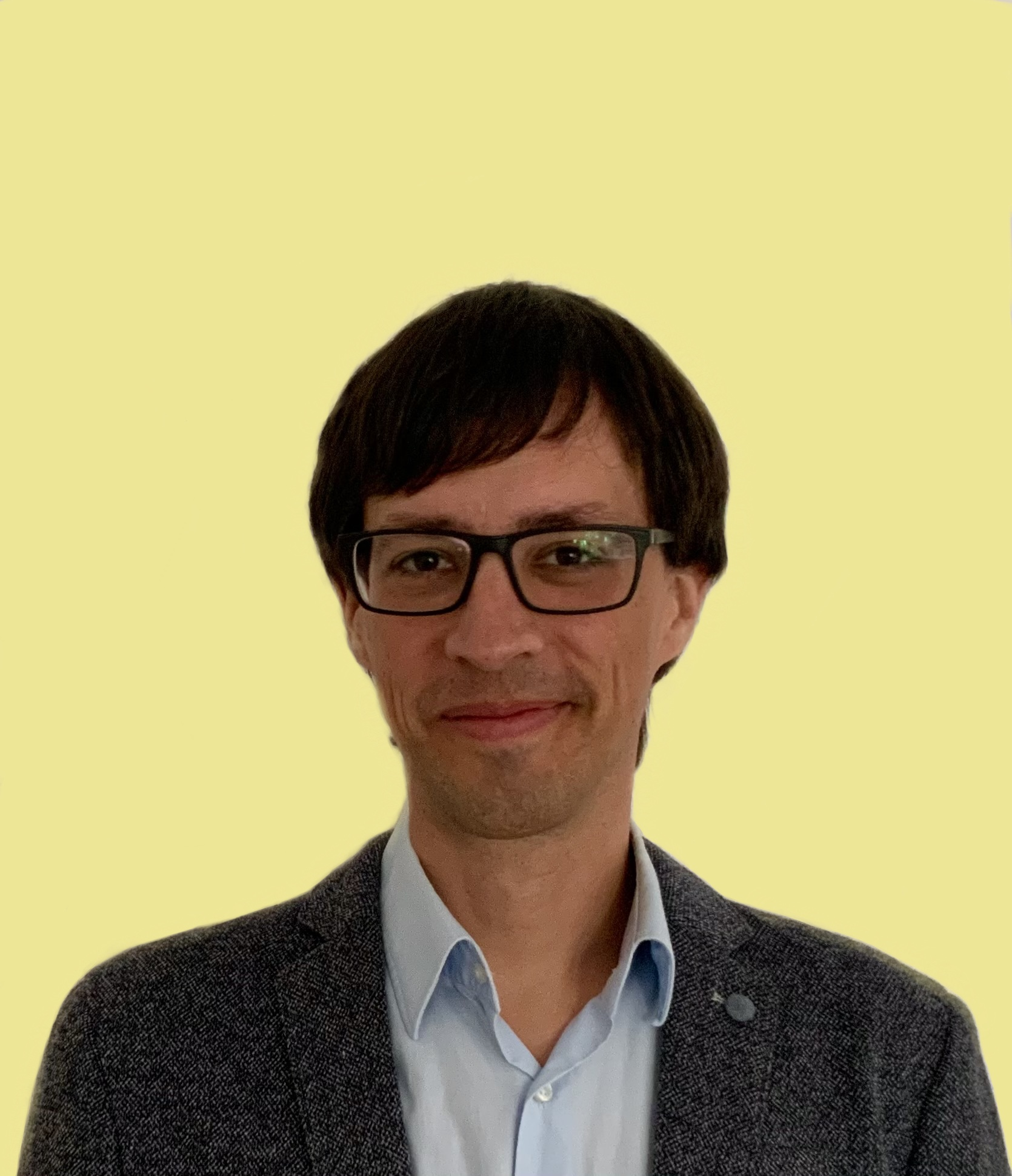Research interest List of publications     - in peer reviewed journals     - monographs     - preprints     - further publications Further publications by students under my supervision My articles on Google Scholar My articles on Math Sci Net My articles on arXiv PI in the SFB-TRR 195 Editor of Analysis Mathematica

## Research interest

 My research is on compact quantum groups. I am working at the intersection of analysis, algebra and combinatorics, with links to free probability. Amongst others, I am primarily interested in symmetry structures within any framework, in particular a noncommutative one, i.e. structures involving noncommutative algebras arising in the context of operators on Hilbert spaces, matrices in linear algebra or observables in quantum physics, just to name a few. I am investigating compact quantum groups from different perspectives, using various methods interfering with many other areas of mathematics such as: Functional analysis: (universal) C*-algebras, von Neumann algebras, K-Theory Algebra: Hopf algebras, automorphisms of graphs, computer algebra Combinatorics: partitions of sets, finite graphs, counting problems Topology, cohomology: noncommutative topology (C*-algebras), K-Theory, (Hochschild) cohomology Quantum symmetries: "easy" quantum groups, noncommutative harmonic analysis, quantum automorphisms of graphs Geometry: noncommutative geometry, notions of symmetry, deformation/quantization Tensor categories: representation theory of quantum groups, Schur-Weyl results Quantum information theory: graph isomorphism games, construction of highly entangled quantum states Stochastical methods: distributional symmetries, free probability, Lévy processes My main expertise is around "easy" quantum groups and the strategy to express analytic, algebraic or representation theoretical properties by purely combinatorial means. What actually is Quantum Symmetry (Oberwolfach Snapshot)?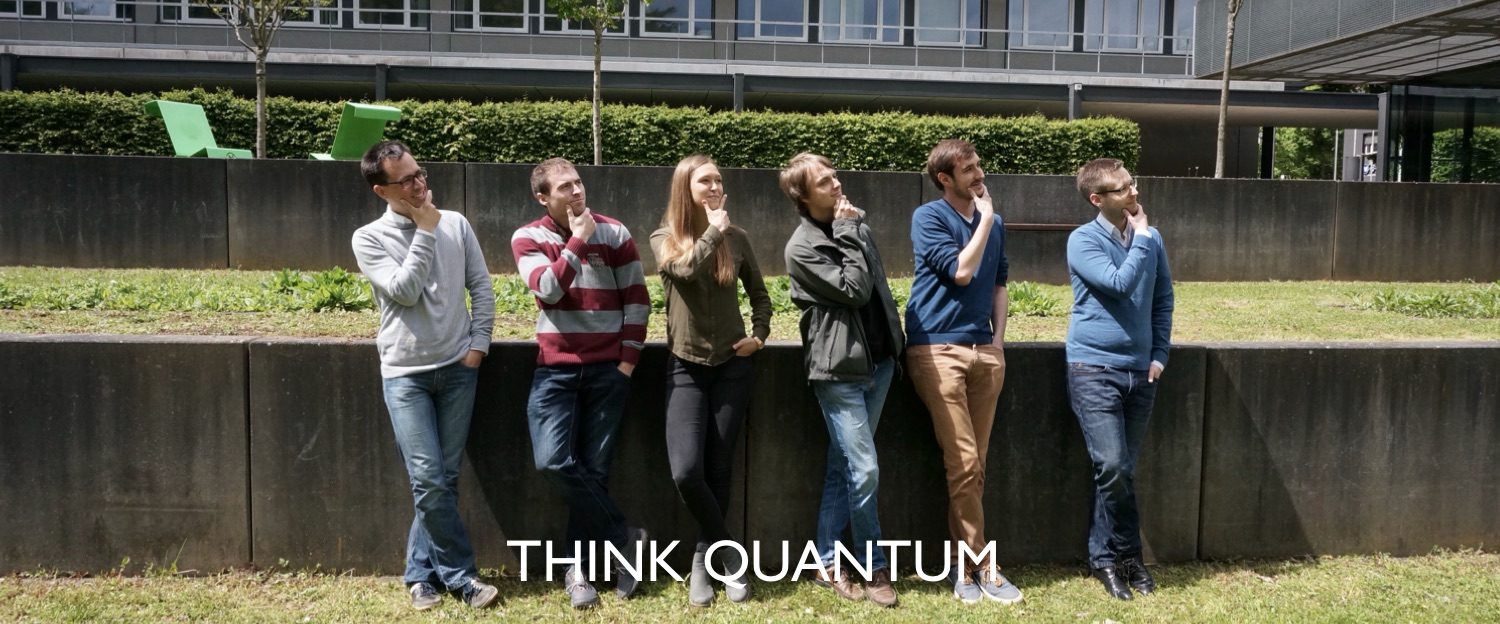Moritz Weber, Stefan Jung, Laura Maaßen, Daniel Gromada, Simon Schmidt, Alexander Mang (May 2019) PhD students

## Member (PI) of the SFB-TRR 195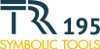Since 1 January 2017, I am one of the PI's of the SFB-TRR 195 Symbolic Tools in Mathematics and their Applications (RWTH Aachen, TU Kaiserslautern, Saarland University, and others), funded by the DFG (German Research Foundation). My project in the first phase (2017-2020) was: I.13 - Computational classification of orthogonal quantum groups. My project in the second phase (2021-2024) is: A25 - Quantum symmetries and quantum isomorphisms of graphs

## Editor of Analysis Mathematica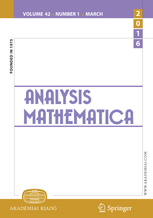Since January 2019, I am an editor of Analysis Mathematica for the area functional analysis, operator algebras and quantum groups. Submissions welcome! Alternative journals with editors coming from operator algebras may be found on a list by Hannes Thiel.

## Publications

### Preprints

Show abstracts

• Brannan, Michael; Eifler, Kari; Voigt, Christian; Weber, M.
Quantum Cuntz-Krieger algebras
arXiv:2009.09466 [math.OA, math.QA], 40 pages (2020).

• Schmidt, Simon; Vogeli, Chase; Weber, M.
Uniformly vertex-transitive graphs
arXiv:1912.00060 [math.CO], 17 pages (2019).

• Eder, Christian; Levandovskyy, Viktor; Schanz, Julien; Schmidt, Simon; Steenpass, Andreas; Weber, M.
Existence of quantum symmetries for graphs on up to seven vertices: a computer based approach
arXiv:1906.12097 [math.QA, math.CO], 15 pages + appendix (2019).

• Weber, M.
Partition C*-algebras
arXiv:1710.06199 [math.OA, math.CO, math.QA], 19 pages + 11 pages of appendix and references (2017).

• Weber, M.
Partition C*-algebras II - links to compact matrix quantum groups
arXiv:1710.08662 [math.OA, math.CO, math.QA], 27 pages (2017).

• Cébron, Guillaume; Weber, M.
Quantum groups based on spatial partitions
arXiv:1609.02321 [math.QA, math.OA], 32 pages (2016).

### Monographs

Show abstracts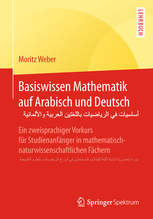Weber, M. Basiswissen Mathematik auf Arabisch und Deutsch Springer Spektrum, 2018 168 pages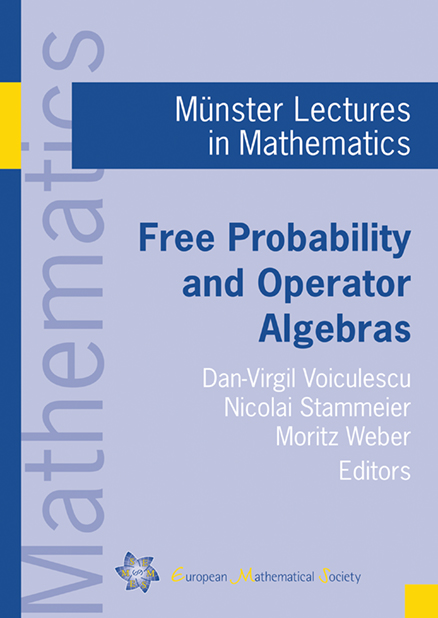Voiculescu, Dan-Virgil; Stammeier, Nicolai; Weber, M. (eds) Free probability and operator algebras Münster Lecture Notes in Mathematics European Mathematical Society (EMS) 132 pagesZürich, 2016

### Chapters in monographs

• Weber, M.
Basics in free probability, 6 pages
in Free probability and operator algebras, ed. by Dan-V. Voiculescu, N. Stammeier, M. Weber, EMS, 2016.

• Weber, M.
Easy quantum groups, 23 pages
in Free probability and operator algebras, ed. by Dan-V. Voiculescu, N. Stammeier, M. Weber, EMS, 2016.

Show abstracts

## Further publications by students under my supervision

 updated: 17 November 2020   Moritz Weber Impressum